#0
0
01. 云栖社区>
2. Java架构沉思录>
3. 博客>
4. 正文

# 前言

●  Executors 线程池工厂能创建哪些线程池
●  如何手动创建线程池
●  如何扩展线程池
●  如何优化线程池的异常信息
●  如何设计线程池中的线程数量

# 1. Executors 线程池工厂能创建哪些线程池

``````
``````
`static class MyTask implements Runnable {`
``` ```
`@Override`
``` ```
`public void run() {`
``` ```
`System.out`
``` ```
`.println(System.currentTimeMillis() + ": Thread ID :" + Thread.currentThread().getId());`
``` ```
`try {`
``` ```
`Thread.sleep(1000);`
``` ```
`} catch (InterruptedException e) {`
``` ```
`e.printStackTrace();`
``` ```
`}`
``` ```
`}`
``` ```
`}`
``` ```

``` ```
` public static void main(String[] args) {`
``` ```
`MyTask myTask = new MyTask();`
``` ```
`ExecutorService service1 = Executors.newFixedThreadPool(5);`
``` ```
`for (int i = 0; i < 10; i++) {`
``` ```
`service1.submit(myTask);`
``` ```
`}`
``` ```
`service1.shutdown();`
``` ```
`}`
``` ```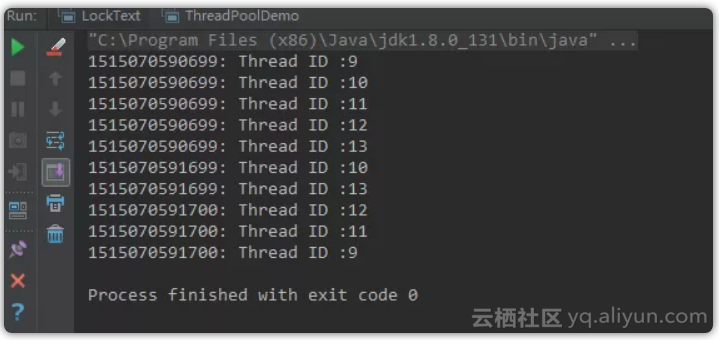1. 固定线程池 ExecutorService service1 = Executors.newFixedThreadPool(5); 该方法返回一个固定线程数量的线程池。该线程池中的线程数量始终不变。

2. 单例线程池 ExecutorService service3 = Executors.newSingleThreadExecutor(); 该方法返回一个只有一个线程的线程池。

3. 缓存线程池 ExecutorService service2 = Executors.newCachedThreadPool(); 该方法返回一个可根据实际情况调整线程数量的线程池，线程池的线程数量不确定。

``````
``````
`class A {`
``` ```
`public static void main(String[] args) {`
``` ```
`ScheduledThreadPoolExecutor service4 = (ScheduledThreadPoolExecutor) Executors`
``` ```
`.newScheduledThreadPool(2);`
``` ```

``` ```
` // 如果前面的任务没有完成，则调度也不会启动`
``` ```
`service4.scheduleAtFixedRate(new Runnable() {`
``` ```
`@Override`
``` ```
`public void run() {`
``` ```
`try {`
``` ```
`// 如果任务执行时间大于间隔时间，那么就以执行时间为准（防止任务出现堆叠）。`
``` ```
`Thread.sleep(10000);`
``` ```
`System.out.println(System.currentTimeMillis() / 1000);`
``` ```
`} catch (InterruptedException e) {`
``` ```
`e.printStackTrace();`
``` ```
`}`
``` ```
`}// initialDelay（初始延迟） 表示第一次延时时间 ; period 表示间隔时间`
``` ```
`}, 0, 2, TimeUnit.SECONDS);`
``` ```

``` ```

``` ```
`service4.scheduleWithFixedDelay(new Runnable() {`
``` ```
`@Override`
``` ```
`public void run() {`
``` ```
`try {`
``` ```
`Thread.sleep(5000);`
``` ```
`System.out.println(System.currentTimeMillis() / 1000);`
``` ```
`} catch (InterruptedException e) {`
``` ```
`e.printStackTrace();`
``` ```
`}`
``` ```
`}// initialDelay（初始延迟） 表示延时时间；delay + 任务执行时间 = 等于间隔时间 period`
``` ```
`}, 0, 2, TimeUnit.SECONDS);`
``` ```

``` ```
`// 在给定时间，对任务进行一次调度`
``` ```
`service4.schedule(new Runnable() {`
``` ```
`@Override`
``` ```
`public void run() {`
``` ```
`System.out.println("5 秒之后执行 schedule");`
``` ```
`}`
``` ```
`}, 5, TimeUnit.SECONDS);`
``` ```
`}`
``` ```
`}}`
``` ```

# 2. 如何手动创建线程池

``````
``````
`/**`
``` ```
`* 默认5条线程（默认数量，即最少数量），`
``` ```
`* 最大20线程（指定了线程池中的最大线程数量），`
``` ```
`* 空闲时间0秒（当线程池梳理超过核心数量时，多余的空闲时间的存活时间，即超过核心线程数量的空闲线程，在多长时间内，会被销毁），`
``` ```
`* 等待队列长度1024，`
``` ```
`* 线程名称[MXR-Task-%d],方便回溯，`
``` ```
`* 拒绝策略：当任务队列已满，抛出RejectedExecutionException`
``` ```
`* 异常。`
``` ```
`*/`
``` ```
`private static ThreadPoolExecutor threadPool = new ThreadPoolExecutor(5, 20, 0L,`
``` ```
`TimeUnit.MILLISECONDS, new LinkedBlockingQueue<>(1024)`
``` ```
`, new ThreadFactoryBuilder().setNameFormat("My-Task-%d").build()`
``` ```
`, new AbortPolicy()`
``` ```
`);`
``` ```

●  corePoolSize 线程池中核心线程数量
●  maximumPoolSize 最大线程数量
●  keepAliveTime 空闲时间（当线程池梳理超过核心数量时，多余的空闲时间的存活时间，即超过核心线程数量的空闲线程，在多长时间内，会被销毁）
●  unit 时间单位
●  workQueue 当核心线程工作已满，需要存储任务的队列

●  handler 当队列满了之后的拒绝策略

●  无界队列： 默认大小 int 最大值，因此可能会耗尽系统内存，引起OOM，非常危险。
●  直接提交的队列 ： 没有容量，不会保存，直接创建新的线程，因此需要设置很大的线程池数。否则容易执行拒绝策略，也很危险。
●  有界队列：如果core满了，则存储在队列中，如果core满了且队列满了，则创建线程，直到maximumPoolSize 到了，如果队列满了且最大线程数已经到了，则执行拒绝策略。
●  优先级队列：按照优先级执行任务。也可以设置大小。

●  AbortPolicy ：直接抛出异常，阻止系统正常工作.
●  CallerRunsPolicy : 只要线程池未关闭，该策略直接在调用者线程中，运行当前被丢弃的任务。显然这样做不会真的丢弃任务，但是，任务提交线程的性能极有可能会急剧下降。

``````
``````
`/**`
``` ```
`* The default thread factory`
``` ```
`*/`
``` ```
`static class DefaultThreadFactory implements ThreadFactory {`
``` ```
`private static final AtomicInteger poolNumber = new AtomicInteger(1);`
``` ```
`private final ThreadGroup group;`
``` ```
`private final AtomicInteger threadNumber = new AtomicInteger(1);`
``` ```
`private final String namePrefix;`
``` ```

``` ```
`DefaultThreadFactory() {`
``` ```
`SecurityManager s = System.getSecurityManager();`
``` ```
`group = (s != null) ? s.getThreadGroup() :`
``` ```
`Thread.currentThread().getThreadGroup();`
``` ```
`namePrefix = "pool-" +`
``` ```
`poolNumber.getAndIncrement() +`
``` ```
`"-thread-";`
``` ```
`}`
``` ```

``` ```
`public Thread newThread(Runnable r) {`
``` ```
`Thread t = new Thread(group, r,`
``` ```
`namePrefix + threadNumber.getAndIncrement(),`
``` ```
`0);`
``` ```
`if (t.isDaemon())`
``` ```
`t.setDaemon(false);`
``` ```
`if (t.getPriority() != Thread.NORM_PRIORITY)`
``` ```
`t.setPriority(Thread.NORM_PRIORITY);`
``` ```
`return t;`
``` ```
`}`
``` ```
`}`
``` ```

# 3. 如何扩展线程池

``````
``````
`/**`
``` ```
`* 如何扩展线程池，重写 beforeExecute, afterExecute, terminated 方法，这三个方法默认是空的。`
``` ```
`*`
``` ```
`* 可以监控每个线程任务执行的开始和结束时间，或者自定义一些增强。`
``` ```
`*`
``` ```
`* 在 Worker 的 runWork 方法中，会调用这些方法`
``` ```
`*/public class ExtendThreadPoolDemo {`
``` ```

``` ```
` static class MyTask implements Runnable {`
``` ```

``` ```
`String name;`
``` ```

``` ```
`public MyTask(String name) {`
``` ```
`this.name = name;`
``` ```
`}`
``` ```

``` ```
`@Override`
``` ```
`public void run() {`
``` ```
`System.out`
``` ```
`.println("正在执行：Thread ID:" + Thread.currentThread().getId() + ", Task Name = " + name);`
``` ```
`try {`
``` ```
`Thread.sleep(100);`
``` ```
`} catch (InterruptedException e) {`
``` ```
`e.printStackTrace();`
``` ```
`}`
``` ```
`}`
``` ```
`}`
``` ```

``` ```

``` ```
`public static void main(String[] args) throws InterruptedException {`
``` ```
`ExecutorService es = new ThreadPoolExecutor(5, 5, 0L, TimeUnit.MILLISECONDS,`
``` ```
`new LinkedBlockingQueue<>()) {`
``` ```
`@Override`
``` ```
`protected void beforeExecute(Thread t, Runnable r) {`
``` ```
`System.out.println("准备执行：" + ((MyTask) r).name);`
``` ```
`}`
``` ```

``` ```
`@Override`
``` ```
`protected void afterExecute(Runnable r, Throwable t) {`
``` ```
`System.out.println("执行完成： " + ((MyTask) r).name);`
``` ```
`}`
``` ```

``` ```
`@Override`
``` ```
`protected void terminated() {`
``` ```
`System.out.println("线程池退出");`
``` ```
`}`
``` ```
`};`
``` ```

``` ```
`for (int i = 0; i < 5; i++) {`
``` ```
`MyTask myTask = new MyTask("TASK-GEYM-" + i);`
``` ```
`es.execute(myTask);`
``` ```
`Thread.sleep(10);`
``` ```

``` ```
`}`
``` ```

``` ```
`es.shutdown();`
``` ```
`}}`
``` ```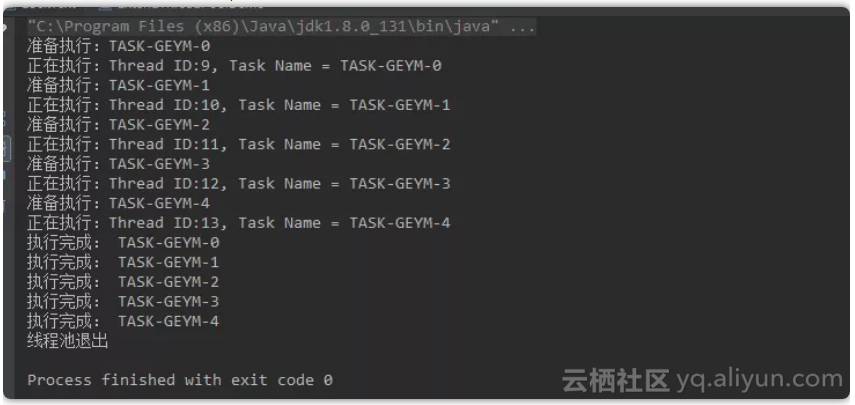# 4. 如何优化线程池的异常信息

``````
``````
`public static void main(String[] args) throws ExecutionException, InterruptedException {`
``` ```

``` ```
`ThreadPoolExecutor executor = new ThreadPoolExecutor(0, Integer.MAX_VALUE, 0L,`
``` ```
`TimeUnit.MILLISECONDS, new SynchronousQueue<>());`
``` ```

``` ```
`for (int i = 0; i < 5; i++) {`
``` ```
`executor.submit(new DivTask(100, i));`
``` ```
`}`
``` ```
`}`
``` ```

``` ```

``` ```
`static class DivTask implements Runnable {`
``` ```
`int a, b;`
``` ```

``` ```
`public DivTask(int a, int b) {`
``` ```
`this.a = a;`
``` ```
`this.b = b;`
``` ```
`}`
``` ```

``` ```
`@Override`
``` ```
`public void run() {`
``` ```
`double re = a / b;`
``` ```
`System.out.println(re);`
``` ```
`}`
``` ```
`}`
``` ```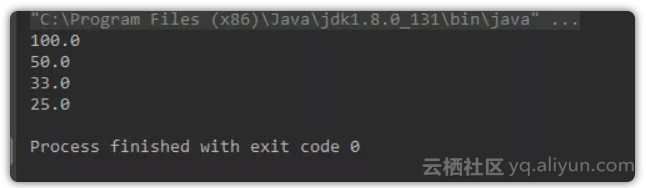``````
``````
`static class TraceThreadPoolExecutor extends ThreadPoolExecutor {`
``` ```

``` ```
` public TraceThreadPoolExecutor(int corePoolSize, int maximumPoolSize, long keepAliveTime,`
``` ```
`TimeUnit unit, BlockingQueue<Runnable> workQueue) {`
``` ```
`super(corePoolSize, maximumPoolSize, keepAliveTime, unit, workQueue);`
``` ```
`}`
``` ```

``` ```
`@Override`
``` ```
`public void execute(Runnable command) {// super.execute(command);`
``` ```
`super.execute(wrap(command, clientTrace(), Thread.currentThread().getName()));`
``` ```
`}`
``` ```

``` ```
`@Override`
``` ```
`public Future<?> submit(Runnable task) {// return super.submit(task);`
``` ```
`return super.submit(wrap(task, clientTrace(), Thread.currentThread().getName()));`
``` ```
`}`
``` ```

``` ```
`private Exception clientTrace() {`
``` ```
`return new Exception("Client stack trace");`
``` ```
`}`
``` ```

``` ```
`private Runnable wrap(final Runnable task, final Exception clientStack,`
``` ```
`String clientThreaName) {`
``` ```
`return new Runnable() {`
``` ```
`@Override`
``` ```
`public void run() {`
``` ```
`try {`
``` ```
`task.run();`
``` ```
`} catch (Exception e) {`
``` ```
`e.printStackTrace();`
``` ```
`clientStack.printStackTrace();`
``` ```
`throw e;`
``` ```
`}`
``` ```
`}`
``` ```
`};`
``` ```
`}`
``` ```
`}`
``` ```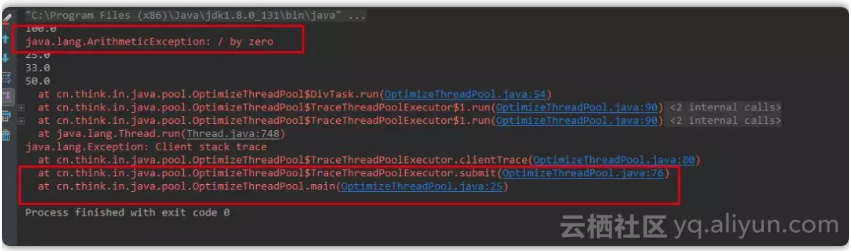# 5. 如何设计线程池中的线程数量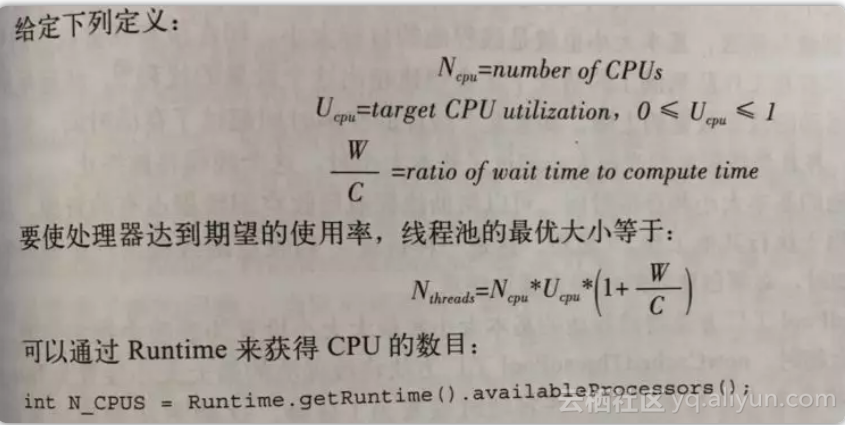Runtime.getRuntime().availableProcessors()；

+ 关注